# 1296 Last Digit Effort

If you always use a calculator to find the roots of perfect powers like 1296, then you will likely miss out on discovering the wonderful patterns those powers have. Look at the table below. What do you notice? What do you wonder?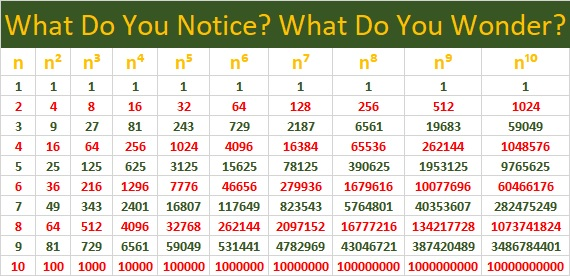When I looked at it, I noticed several patterns and I wondered about a few things that I explored to see if they were true. However, I won’t tell you what I noticed or what conjectures I made until the end of this post, because I don’t want to spoil YOUR chance to discover those patterns yourself. I will tell you that there are MANY patterns in the table above and that most of my conjectures were true, but one of them turned out not to be.

Besides the patterns that you will have to discover for yourself, the table can be very useful: You can use the table to find several fractional powers of the numbers in the body of the table. For example, if you wanted to find out what is 1296^(7/4), then you could simply run your finger down the n⁷ column and the n⁴ column simultaneously until you reach 1296 on the n⁴ column (because 4 is the denominator of the fractional power). Your answer, 279936, will be in the n⁷ column on the same row as 1296.

However, if you wanted to find 1296^(3/2), you would either have to expand the table to include 36² and 36³, or you would have to use the fact that 3/2 = 6/4. Then you could use the process of the previous paragraph to see that
1296^(3/2) = 1296^(6/4) = 46656.

Now I’ll share some more facts about the number 1296:

• 1296 is a composite number, a perfect square, and a perfect fourth power.
• Prime factorization: 1296 = 2 × 2 × 2 × 2 × 3 × 3 × 3 × 3, which can be written 1296 = 2⁴ × 3⁴
• The exponents in the prime factorization are 4 and 4. Adding one to each and multiplying we get (4 + 1)(4 + 1) = 5 × 5 = 25. Therefore 1296 has exactly 25 factors.
• Factors of 1296: 1, 2, 3, 4, 6, 8, 9, 12, 16, 18, 24, 27, 36, 48, 54, 72, 81, 108, 144, 162, 216, 324, 432, 648, 1296
• Factor pairs: 1296 = 1 × 1296, 2 × 648, 3 × 432, 4 × 324, 6 × 216, 8 × 162, 9 × 144, 12 × 108, 16 × 81, 18 × 72, 24 × 54, 27 × 48, or 36 × 36
• Taking the factor pair with the largest square number factor, we get √1296 = (√36)(√36) = 36.1296 is the smallest number with exactly 25 factors.

There are MANY different factor trees you could make for 1296, but here are some made with squares: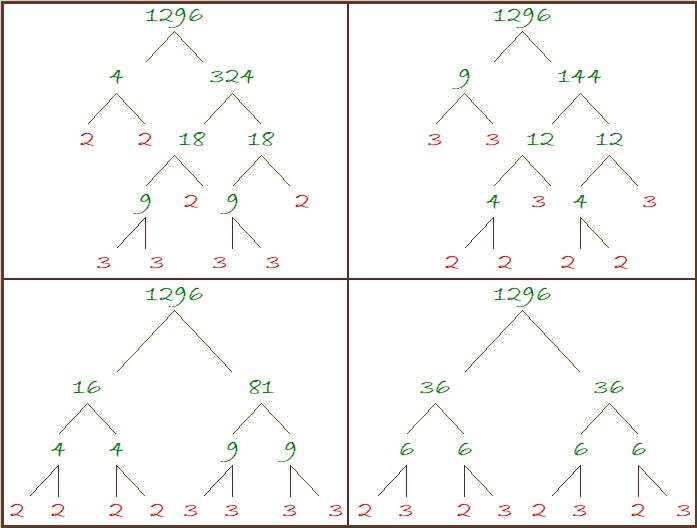1296 is the 21st Friedman number because of its digits and 9²×16 = 1296. (The same digits are on both sides of that equation.)

Since 36 is a triangular number and 36² = 1296, we get this wonderful, powerful fact:
(1 + 2 + 3 + 4 + 5 + 6 + 7 + 8)² = 1³ + 2³ + 3³ + 4³ + 5³ + 6³ + 7³ + 8³ = 1296

1296 looks interesting and often rather square when it is written in several bases:
It’s1210000 in BASE 3,
10000 in BASE 6,
2420 in BASE 8,
1700 in BASE 9,
1296 in BASE 10
900 in BASE 12,
789 in BASE 13 (Ha, ha. Seven ate nine!),
484 in BASE 17,
400 in BASE 18,
169 in BASE 33,
144 in BASE 34,
121 in BASE 35, and
100 in BASE 36

Do you know the square roots in base 10 of all the numbers in bold? Indeed, 1296 is a fascinating square number!

Here are some things I noticed on the table of powers for numbers ending in . . .

• 1: The last digit is always 1
• 2: The pattern for the last digit is 2, 4, 8, 6, repeating
• 3: The pattern for the last digit is 3, 9, 7, 1, repeating
• 4: The pattern for the last digit is 4, 6, 4, 6, repeating
• 5: The last digit is always 5
• 6: The last digit is always 6
• 7: The pattern for the last digit is 7, 9, 3, 1, repeating (3’s pattern backward but starting with 7)
• 8: The pattern for the last digit is 8, 4, 2, 6, repeating (2’s pattern backward but starting with 8)
• 9: The pattern for the last digit is 9, 1, 9, 1, repeating
• 0: The last digit is always 0

For the squares:

• 1² and 9² both end in 1, and 1 + 9 = 10
• 2² and 8² both end in 4, and 2 + 8 = 10
• 3² and 7² both end in 9, and 3 + 7 = 10
• 4² and 6² both end in 6, and 4 + 6 = 10

For the cubes:

• 1³ ends in 1, and 9³ ends in 9
• 2³ ends in 8, and 8³ ends in 2; 2 + 8 = 10
• 3³ ends in 7, and 7³ ends in 3; 3 + 7 = 10
• 4³ ends in 4, and 6³ ends in 6

Other observations:

• No matter what n is, the last digit of n, n⁵, and n⁹ are always the same. Yes, that pattern continues with n¹³ and so forth.
• The number of digits for 10ᵃ is a+1, and the number of digits for 9ᵃ is a.

Dan Bach noticed something else.

I had never heard of Benford’s Law before, so I’ve learned something new here!  All of us can learn something new by observing patterns and listening to each other.

# 1225 is a Triangular Number, a Perfect Square, and . . .

1 and 36 are both triangular numbers and square numbers. The next number that can make the same claim is 1225. Why is this so?

1225 is a triangular number that just happens to be the sum of two consecutive triangular numbers. Two consecutive triangular numbers can always be made into a perfect square. Here’s a gif that tries to illustrate these facts about 1225: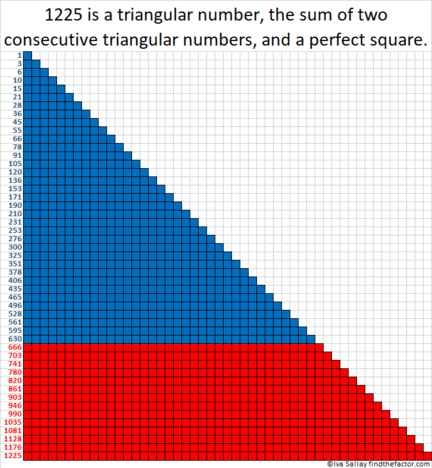GIFs like this at MakeaGif

1225 is the 49th triangular number because 49(50)/2 =1225

It is the 35th square number because 35² = 1225

That it is both a triangular number AND a square number is pretty remarkable. But guess what? 1225 is ALSO a hexagonal number.

1225 is the 25th hexagonal number because (25)(2·25 – 1)= 1225
In fact, 1225 is the smallest number greater than 1 that is a triangle, a square, AND a hexagon! (Yeah, 1 is also all three and a whole more, but does 1 dot REALLY look like a triangle, a square, a hexagon and everything else all at the same time?)
All of these facts are great reasons to get very excited about the number 1225. Here are some more facts about this number:
• 1225 is a composite number.
• Prime factorization: 1225 = 5 × 5 × 7 × 7, which can be written 1225 = 5²× 7²
• The exponents in the prime factorization are 2 and 2. Adding one to each and multiplying we get (2 + 1)(2 + 1) = 3 × 3 = 9. Therefore 1225 has exactly 9 factors.
• Factors of 1225: 1, 5, 7, 25, 35, 49, 175, 245, 1225
• Factor pairs: 1225 = 1 × 1225, 5 × 245, 7 × 175, 25 × 49, or 35 × 35
• 1225 is a perfect square. √1225 = 35
Not only is 1225 a triangular number that is the sum of two other triangular numbers, but
1225 is also a square that is the sum of two other squares!
28² + 21² = 35² = 1225

1225 is the hypotenuse of two Pythagorean triples:
735-980-1225 which is (3-4-5) times 245
343-1176-1225 calculated from 28² – 21², 2(28)(21), 28² + 21²,
but it is also (7-24-25) times 49

1225 looks like a square in some other bases:
It’s 441 in BASE 17,
169 in BASE 32,
144 in BASE 33,
121 in BASE 34, and
100 in BASE 35

Stetson.edu reports that 1225 is the smallest number that can be written as the sum of four cubes three different ways. It looks like these are the three ways:
10³ + 6 ³ + 2³ + 1³ = 1225
9³ + 6³ + 6³ + 4³ = 1225
8³ + 7³ + 7³ + 3³ = 1225

1225 is, indeed, the smallest number that has all the special properties listed above!

# You’ll Be Impressed By 841’s Number Facts

841 is a very cool square number. 29 × 29 = 841. You can write it more compactly: 29² = 841. Here are some facts about this square number:

841 is the sum of the 29th and the 28th triangular numbers because 841 = 435 + 406.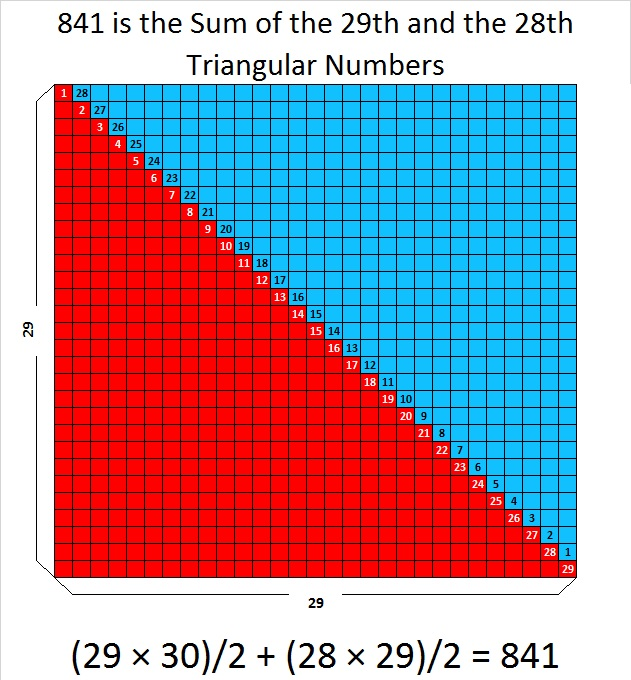What’s that you say? Every other square number can make a similar claim? Oh. … Wait a minute…Not EVERY square number can do that. One is a square number, and it’s NOT the sum of two triangular numbers. So there…

How about this… 841 is the sum of the first 29 odd numbers. That makes it the sum of all the odd numbers from 1 to 57. That’s impressive!What? All square numbers do that? Huh??? n² is always the sum of the first n odd numbers? Why’d you have to tell me that fact isn’t particularly unique either!

Okay…841 is an interesting number in a few other bases. Pay attention….  841 is

• 100 in BASE 29
• 121 in BASE 28
• 144 in BASE 27
• 169 in BASE 26
• 441 in BASE 14

Ha! You didn’t know that one! … Now don’t go spoiling my glee by telling me that those same square numbers show up for a few other square numbers, too. Don’t tell me that!

Give me one more chance to impress you….This square number, 841, is the sum of two consecutive square numbers, so 20² + 21² = 841 = 29². That hasn’t happened to a square number since 3² + 4² = 25 = 5². That makes 841 the 21st Centered Square Number but only the SECOND square number that is both kinds of squares! There may be an infinite number of squares that do the same thing, but it is still a fairly unique characteristic.There are 441 blue squares in that graphic. There are 400 squares that are orange, red, green, purple, or black. 441 + 400 = 841.

Here are a few other tidbits about 841:

841 is the sum of the nine prime numbers from 73 to 109. It is also the sum of three consecutive primes: 277 +  281 + 283 = 841

841 is the hypotenuse of TWO Pythagorean triples:

• 580-609-841 which is 29 times (20-21-29)
• 41-840-841 calculated from 21² – 20², 2(21)(20), 21² + 20²

841 Pythagorean triple Recursion: Hmm…How Quickly Can I Give You a Headache? Warning! Reading this set of bullet points might overload your brain:

• 5² + 2² = 29, so (2∙5∙2)² + (5² – 2²)² = (5² + 2²)² which means 20² + 21² = 29² = 841.
• 21² + 20² = 841, so (2∙21∙20)² + (21² – 20²)² = (21² + 20²)² which means 840² + 41² = 841² = (29²)² = 707281.
• 840² + 41² = 707281, so (2∙840∙41)² + (840² – 41²)² = (840² + 41²)² which means 68880² + 703919² = 707281² = ((29²)²)² = 500,246,412,961
• We could go on forever with even bigger powers of 29 …

We’ll finish with just some simple, easy-on-the-brain facts about 841:

29 is the tenth prime number. Its square, 841, is only the tenth number to have exactly three factors.

• 841 is a composite number.
• Prime factorization: 841 = 29²
• The exponent in the prime factorization is 2. Adding one we get (2 + 1) = 3. Therefore 841 has exactly 3 factors.
• Factors of 841: 1, 29, 841
• Factor pairs: 841 = 1 × 841 or 29 × 29
• 841 is a perfect square. √841 = 29I’m impressed by all this, even if you aren’t.

# 441 Consecutive Numbers

The first 6 triangular numbers are

• 1 = 1
• 1 + 2 = 3
• 1 + 2 + 3 = 6
• 1 + 2 + 3 + 4 = 10
• 1 + 2 + 3 + 4 + 5 = 15
• 1 + 2 + 3 + 4 + 5 + 6 = 21

For millennia mathematicians have thought triangular numbers were quite interesting. 21 is a triangular number and 21 x 21 = 441 which makes 441 interesting, too. But wait, there’s something else that is very interesting about triangular numbers: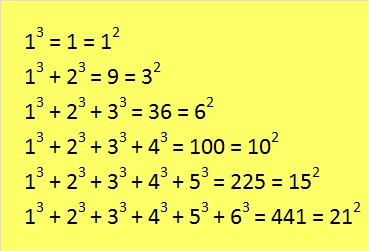It is amazing that when we begin with 1 cube, the sum of n consecutive cubes equals the nth triangular number squared every time!

What  follows is less amazing, but very practical. We can add consecutive numbers several ways to get 441. To find those ways we need to know the factors of 441.

• 441 is a composite number.
• Prime factorization: 441 = 3 x 3 x 7 x 7, which can be written 441 = (3^2) x (7^2)
• The exponents in the prime factorization are 2 and 2. Adding one to each and multiplying we get (2 + 1)(2 + 1) = 3 x 3 = 9. Therefore 441 has exactly 9 factors.
• Factors of 441: 1, 3, 7, 9, 21, 49, 63, 147, 441
• Factor pairs: 441 = 1 x 441, 3 x 147, 7 x 63, 9 x 49, or 21 x 21
• 441 is a perfect square. √441 = 21Because all of the factors for 441 are odd numbers, it is so easy to find consecutive numbers whose sum equal 441. Check out all of these:If we allowed negative numbers in the list of consecutive numbers we could also see that 441 equals the sum of 147 consecutive numbers that are centered around the number 3, and 49 consecutive numbers that are centered around the number 7, and 63 consecutive numbers that are centered around the number 9. All of those sums would be quite long.

Here are some more reasonable-length sums using only consecutive ODD numbers.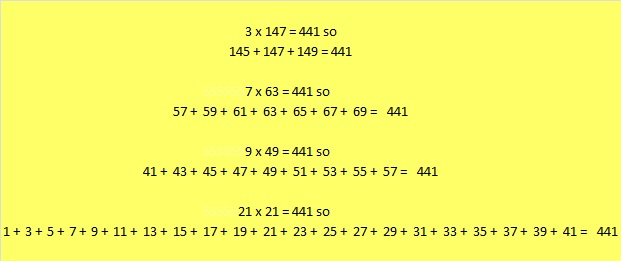That last sum reminds us that we always get n squared when we begin with one and add n consecutive odd numbers together.

# 306 and Level 4

• 306 is a composite number.
• Prime factorization: 306 = 2 x 3 x 3 x 17, which can be written 306 = 2 x 3² x 17
• The exponents in the prime factorization are 1, 2, and 1. Adding one to each and multiplying we get (1 + 1)(2 + 1)(1 + 1) = 2 x 3 x 2 = 12. Therefore 306 has exactly 12 factors.
• Factors of 306: 1, 2, 3, 6, 9, 17, 18, 34, 51, 102, 153, 306
• Factor pairs: 306 = 1 x 306, 2 x 153, 3 x 102, 6 x 51, 9 x 34, or 17 x 18
• Taking the factor pair with the largest square number factor, we get √306 = (√9)(√34) = 3√34 ≈ 17.493Happy Thanksgiving!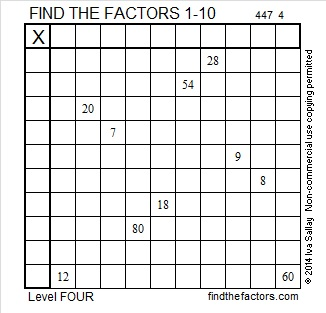Print the puzzles or type the factors on this excel file: 10 Factors 2014-11-24

Here’s a little more about the number 306:

306 = 17 × 18, which means it is the sum of the first 17 even numbers.

• Thus, 2 + 4 + 6 + 8 + . . .  + 30 + 32 + 34 = 306

It also means that we are halfway between 17² and 18², or halfway between 289 and 324. The average of those two numbers is 306.5.

AND it means that 17² + 18² – 1 = 2(306) = 2(17 × 18)# 305 and Level 3

• 305 is a composite number.
• Prime factorization: 305 = 5 x 61
• The exponents in the prime factorization are 1 and 1. Adding one to each and multiplying we get (1 + 1)(1 + 1) = 2 x 2 = 4. Therefore 305 has exactly 4 factors.
• Factors of 305: 1, 5, 61, 305
• Factor pairs: 305 = 1 x 305 or 5 x 61
• 305 has no square factors that allow its square root to be simplified. √305 ≈ 17.464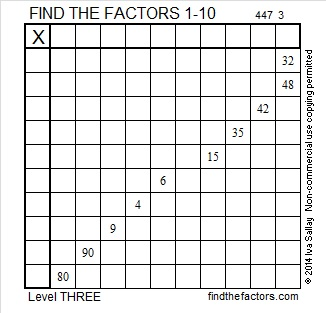Print the puzzles or type the factors on this excel file: 10 Factors 2014-11-24

A Logical Approach to FIND THE FACTORS: Find the column or row with two clues and find their common factor. Write the corresponding factors in the factor column (1st column) and factor row (top row).  Because this is a level three puzzle, you have now written a factor at the top of the factor column. Continue to work from the top of the factor column to the bottom, finding factors and filling in the factor column and the factor row one cell at a time as you go.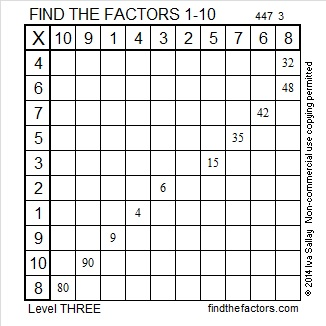# 304 and Level 2

• 304 is a composite number.
• Prime factorization: 304 = 2 x 2 x 2 x 2 x 19, which can be written 304 = (2^4) x 19
• The exponents in the prime factorization are 4 and 1. Adding one to each and multiplying we get (4 + 1)(1 + 1) = 5 x 2 = 10. Therefore 304 has exactly 10 factors.
• Factors of 304: 1, 2, 4, 8, 16, 19, 38, 76, 152, 304
• Factor pairs: 304 = 1 x 304, 2 x 152, 4 x 76, 8 x 38, or 16 x 19
• Taking the factor pair with the largest square number factor, we get √304 = (√16)(√19) = 4√19 ≈ 17.436This 1 – 10 multiplication table has only twelve clues. Can you still complete the entire table?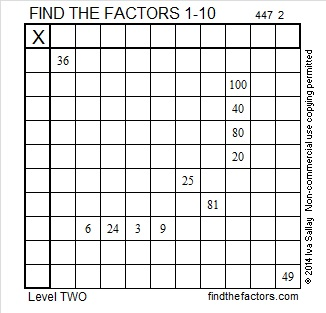Print the puzzles or type the factors on this excel file: 10 Factors 2014-11-24# 303 and Level 1

• 303 is a composite number.
• Prime factorization: 303 = 3 x 101
• The exponents in the prime factorization are 1 and 1. Adding one to each and multiplying we get (1 + 1)(1 + 1) = 2 x 2 = 4. Therefore 303 has exactly 4 factors.
• Factors of 303: 1, 3, 101, 303
• Factor pairs: 303 = 1 x 303 or 3 x 101
• 303 has no square factors that allow its square root to be simplified. √303 ≈ 17.407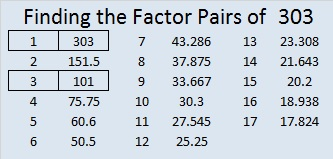Print the puzzles or type the factors on this excel file: 10 Factors 2014-11-24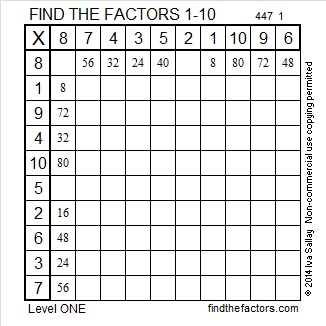# 302 and Level 6

• 302 is a composite number.
• Prime factorization: 302 = 2 x 151
• The exponents in the prime factorization are 1 and 1. Adding one to each and multiplying we get (1 + 1)(1 + 1) = 2 x 2 = 4. Therefore 302 has exactly 4 factors.
• Factors of 302: 1, 2, 151, 302
• Factor pairs: 302 = 1 x 302 or 2 x 151
• 302 has no square factors that allow its square root to be simplified. √302 ≈ 17.378Here’s a very tricky level 6 puzzle to try.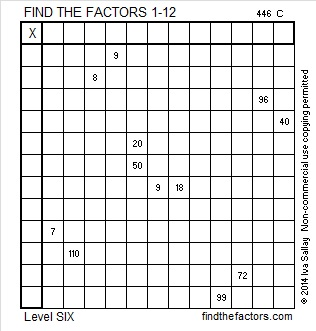Print the puzzles or type the factors on this excel file: 12 Factors 2014-11-17

The logic for this one is complicated. Here is an explanation that may be helpful:

9 is a clue two times in this puzzle. At most one of those 9’s can be 3 x 3. Clue 99 and the other nine will use both 9’s so 72 cannot be 8 x 9 in this puzzle. Here is an explanation of why 72 must be 6 x 12 with the 6 in the first column and the 12 in the top row.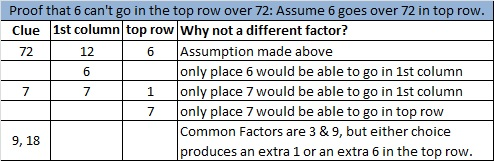Now we can find the rest of the factors easily using logic: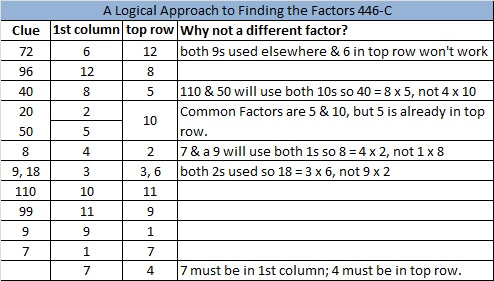# 301 and Level 5

• 301 is a composite number.
• Prime factorization: 301 = 7 x 43
• The exponents in the prime factorization are 1 and 1. Adding one to each and multiplying we get (1 + 1)(1 + 1) = 2 x 2 = 4. Therefore 301 has exactly 4 factors.
• Factors of 301: 1, 7, 43, 301
• Factor pairs: 301 = 1 x 301 or 7 x 43
• 301 has no square factors that allow its square root to be simplified. √301 ≈ 17.349Print the puzzles or type the factors on this excel file: 12 Factors 2014-11-17